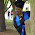## Thursday, October 9, 2014

### Dividing Fractions: Going Beyond Procedures and Learning for Deep Understanding

Having a firm understanding of what a math concept means is just as important if not more than knowing the steps that should be followed in order to solve a math problem.

By drawing step-by-step diagrams in math that show the progression of how numbers or fractional parts change, students are better able to understand math concepts.  Let us look at an example.

Megan read 3/5 of her book in 6 days.  If she read the same number of pages each day, what fraction of the book did she read each day?

For Task Cards That Teach And Review How To Divide Fractions Click Here

To Access Math Task Cards That Teach And Review A Variety Of Math Concepts Click Here

Being able to visualize math concepts helps students understand word problems, and it also helps students have the needed skills in order to solve math problems that are worded in different ways.

#### 1 comment:

1.Thank you for this. It has eased my anxiety on how teach my students